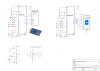# Share My CreationSD Sound Recorder

A sound recorder and playback for Arduino Uno or Nano with ATMEGA328P device using SD card. The card is initialized by the rSD lib and then the code reset the ATMEGA SPI to the code settings. The code uses commands for multi sector reading and writing that don't exist in the rSD library.
The firmware works for SD or SDHC cards only. If you use an SD card (up to 2GB) link PIN2 of the Arduino to Ground.
The recording doesn't use any file system, it uses absolute memory addresses (raw). You can't see files on your computer.
The ADC sample the sound at a rate of 10Ks/s, the low byte is stored to the SD card. In play the bytes are streamed to Timer 0 PWM running at frequency of 64KHz. The 0.65V bias to the ADC input is for setting the signal to the low byte of the converter output. Audio input is 1Vp-p , you can use the mic circuit or another source.
I added a simple 2 transistors amplifier to boost the power to drive 32 Ohm speaker or headphones.
The quality of the audio is reasonable.
The schematic gives the option to wire an SD or a module with micro SDHC.
B4X:
``````Sub Process_Globals

Public Serial1 As Serial
Private tmr As Timer
Private sd As SD
Private spires, spidata As Byte
Private CS As Pin
Private miso As Pin
Private mosi As Pin    'mosi
Private clk As Pin
Private errorLed As Pin
Private recLed As Pin
Private Stop, Play, Pause, Rec As Pin
Private mic, audio, card As Pin
Private card As Pin
Private sdhc=False As Boolean    'standard sd
Private loc As ULong    'location
Private lbyte=0, rbyte As Byte    '
Private stopPB=True, playPB=True, recPB=True, pausePB=True  As Boolean 'pushbutton status

End Sub

Private Sub AppStart
Serial1.Initialize(115200)
sd.Initialize(10) '10 is the CS pin
tmr.Initialize("tmr_Tick", 100)
tmr.Enabled = True
Log("AppStart")
CS.Initialize(10, CS.MODE_OUTPUT)
miso.Initialize(12, miso.MODE_INPUT)
clk.Initialize(13, clk.MODE_OUTPUT)
mosi.Initialize(11, mosi.MODE_OUTPUT)
errorLed.Initialize(9, errorLed.MODE_OUTPUT)
recLed.Initialize(8, recLed.MODE_OUTPUT)
Stop.Initialize(Stop.A2, Stop.MODE_INPUT_PULLUP)
Play.Initialize(Play.A3, Play.MODE_INPUT_PULLUP)
Pause.Initialize(Pause.A5, Pause.MODE_INPUT_PULLUP)
Rec.Initialize(Rec.A4, Rec.MODE_INPUT_PULLUP)
audio.Initialize(6, audio.MODE_OUTPUT)
mic.Initialize(mic.A0, mic.MODE_INPUT)
card.Initialize(2, card.MODE_INPUT_PULLUP)

RunNative ("set_spi",Null)
End Sub

Sub tmr_Tick
'the pushbuttons
If recPB=False Then write
If stopPB=False Then
If sdhc Then loc = 10 Else loc = 5120
End If
End Sub

Sub spi(data As Byte)As Byte    'send byte over spi
spidata=data
RunNative ("spi",Null)
Return spires
End Sub

Sub Command(frame1 As Byte, adrs As ULong, frame2 As Byte)As Byte
Dim i, res As Byte

spi(0xFF)
spi(Bit.And(0x7F,Bit.Or(0x40,frame1)))
spi( Bit.Or(1,frame2))    'CRC And last Bit 1

For i=0 To 9
res = spi(0xFF)
If res <> 0xFF Then Exit
Next
Return res
End Sub

Dim i As UInt
CS.DigitalWrite(False)
If ret <> 0 Then errorLed.DigitalWrite(True)    'If Command failed

Do While stopPB=True And pausePB=True
Do While spi(0xFF) <> 0xFE    'wait For first byte
Loop
For i=0 To 511
rbyte=spi(0xFF)
DelayMicroseconds(65)
Next
spi(0xFF)
If sdhc Then loc=loc + 1 Else loc=loc + 512    'mark location address For Return after Pause
Loop
Command(12,0x00,0xFF)    'Stop transmit
spi(0xFF)
spi(0xFF)
CS.DigitalWrite(True)

End Sub

Sub write    'record
Dim i As UInt
Dim r As Byte

CS.DigitalWrite(False)
recLed.DigitalWrite(True)

r = Command(25,loc,0xFF)    'multi sector write
If r<>0 Then
errorLed.DigitalWrite(True)
recLed.DigitalWrite(False)
End If
spi(0xFF)
spi(0xFF)
spi(0xFF)

Do While stopPB=True And pausePB=True
spi(0xFC)    'multi sector token byte

For i=0 To 511    'stream 512 bytes from ADC To build a sector
'RunNative ("sd_rec",Null)    'comment this line To disable play While recording
spi(lbyte)
'DelayMicroseconds(40)
Next

spi(0xFF) 'CRC
spi(0xFF) 'CRC

For i=0 To 1000
If Bit.And(0x0F,spi(0xFF)) = 0x05 Then    Exit'test If data accepted
Next
If i>998 Then errorLed.DigitalWrite(True)

Do While spi(0xFF) <> 0xFF    'While card busy To write sector
Loop
If sdhc Then loc=loc + 1 Else loc=loc + 512    'mark location address For Return after Pause
Loop
spi(0xFD)    ''stop transfer' token byte is needed to stop multi-sector

spi(0xFF)
spi(0xFF)
Do While spi(0xFF) <> 0xFF    ' While busy
Loop
CS.DigitalWrite(True)
recLed.DigitalWrite(False)
End Sub

#if C
void sd_rec(B4R::Object* o)
{
OCR0A=b4r_main::_lbyte;         //
}
#End if

#if C
{
OCR0A=b4r_main::_rbyte;    //write byte To PWM
}
#End if

#if C
void set_spi(B4R::Object* o)
{
//spi init
SPCR = _BV(SPE) | _BV(MSTR) | _BV(SPR0);  // Enable SPI, Master, set clock rate fck/64 | _BV(SPR1)
SPSR = _BV(SPI2X);    //set clock rate fck/64 = 250KHz

//PWM Timer0
OCR0A = 64;
TCCR0A = _BV(COM0A1) | _BV(WGM01) | _BV(WGM00);  //output in phase, fast PWM mode
TCCR0B = _BV(CS00); // 16MHz/256 = 64KHz

}
#End if

#if C
void spi(B4R::Object* o)
{
SPDR = b4r_main::_spidata;  // Start transmission
while (!(SPSR & _BV(SPIF)));  // Wait For transmission To complete
b4r_main::_spires = SPDR;    // received byte
}
#End if``````

#### Attachments

• sd_lib.zip
2.3 KB · Views: 182
•arduino_sd_b4r.png
33.9 KB · Views: 2,014
Last edited:

B4R Question SD library memory leak
Replies
16
Views
693
B4R Library rSD32 library
Replies
3
Views
1K
B4R Tutorial SD cards
Replies
7
Views
8K